# A nowhere-zero point in a linear mapping

 Importance: High ✭✭✭
 Author(s): Jaeger, Francois
 Subject: Combinatorics » Matrices
 Keywords: invertible nowhere-zero flow
 Recomm. for undergrads: no
 Prize: none
 Posted by: mdevos on: March 8th, 2007
Conjecture   Ifis a finite field with at least 4 elements and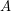is an invertiblematrix with entries in, then there are column vectorswhich have no coordinates equal to zero such that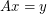.

The motivation for this problem comes from the study of nowhere-zero flows on graphs. Ifis the directed incidence matrix of a graph, then a nowhere-zero-flow onis precisely a vectorso thathas all entries nonzero, and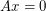. The above conjecture is similar, but is for general (invertible) matrices. Alon and Tarsi have resolved this conjecture for all fields not of prime order using their polynomial technique.

Definition: Say that amatrixis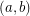-choosable if for allwithand for all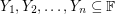with, there exists a vector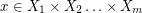and a vectorso that. Note that every matrix is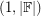-choosable, but that anmatrix is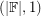-choosable if and only if it is invertible.

Alon and Tarsi actually prove a stronger property than Jaeger conjectured for fields not of prime order. They prove that ifhas characteristic, then every invertible matrix overis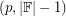-choosable. This result has been extended by DeVos [D] who showed that every such matrix is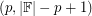-choosable. Yang Yu [Y] has verified that the conjecture holds formatrices with entries inwhen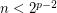.

Jaeger's conjecture is true in a very strong sense for fields of characteristic 2. DeVos [D] proved that every invertible matrix over such a field is-choosable for every. The following conjecture asserts that invertible matrices over fields of prime order have choosability properties nearly as strong.

Conjecture   [The choosability inconjecture (DeVos)] Every invertible matrix with entries infor a primeis-choosable for every.

This is essentially the strongest choosability conjecture one might hope to be true over fields of prime order. I (M. DeVos) don't have any experimental evidence for this at all, so it could be false already for some small examples. However, I suspect that if The permanent conjecture is true, that this conjecture should also be true. In any case, I (M. DeVos) am offering a bottle of wine for this conjecture.

## Bibliography

[A] N. Alon, Combinatorial Nullstellensatz, Combinatorics Probability and Computing 8 (1999) no. 1-2, 7-29. MathSciNet

[AT] N. Alon, M. Tarsi, A Nowhere-Zero Point in Linear Mappings, Combinatorica 9 (1989), 393-395. MathSciNet

[BBLS] R. Baker, J. Bonin, F. Lazebnik, and E. Shustin, On the number of nowhere-zero points in linear mappings, Combinatorica 14 (2) (1994), 149-157. MathSciNet

[D] M. DeVos, Matrix Choosability, J. Combinatorial Theory, Ser. A 90 (2000), 197-209. MathSciNet

[Y] Y. Yu, The Permanent Rank of a Matrix, J. Combinatorial Theory Ser. A 85 (1999), 237-242. MathSciNet

* indicates original appearance(s) of problem.